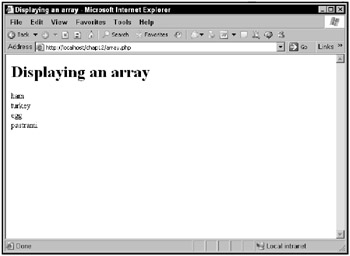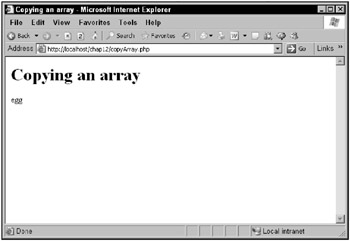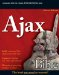Working with Arrays

The next step up in PHP data handling from simple variables is to work with arrays, which are collections of data items stored under a single name, just as in JavaScript. Each element in an array can be addressed easily, by index.

Creating arrays

It’s easy to create arrays in PHP. Just as you can assign values to variables to create those variables, so you can assign values to the elements of an array to create that array. PHP knows that if you use the array index operator [] after a variable’s name, you’re working with an array.

For example, take a look at this PHP statement:

\$sandwiches = "ham";

This statement actually creates an array named \$sandwiches and sets the element at index 1 to ham. From now on, you can refer to this element as you would any simple variable; you just use the index value to make sure you reference the data you want, like this:

echo \$sandwiches;

This statement would echo ham. You can add new values with different numeric indexes:

\$sandwiches = "turkey"; \$sandwiches = "roast beef";

Now you can refer to \$sandwiches (which is ham), \$sandwiches (which is turkey), and \$sandwiches (which is roast beef).

And, of course, you can store numeric values as well:

\$sandwiches = 200; \$sandwiches = 300;

So far, the code you’ve seen has stored strings using numeric indexes, but, interestingly, you can also use string indexes. Here’s an example:

\$sandwich_inventory["Pittsburgh"] = 2343;  \$sandwich_inventory["Albany"] = 5778;  \$sandwich_inventory["Houston"] = 18843;

You can refer to the values in this array by string, as \$sandwich_inventory["Pittsburgh"] (which holds 2343), \$sandwich_inventory["Albany"] (which holds 5778), and \$sand-wich_inventory["Houston"] (which holds 18843). In fact, in PHP, the same array can use both numeric and text indexes.

You can also create arrays with the array statement. Here’s an example showing how that works:

\$data = array(15, 18, 22);

This creates an array named \$data, with the contents 15, 18, 22.

By default, PHP starts the numbering of array elements with 0. What if you wanted to start with an index value of 1? You could specify that with the => operator like this:

\$sandwiches = array(1 => "ham", "turkey", "roast beef");

Now the array would look like this:

\$sandwiches = "ham";  \$sandwiches = "turkey"; \$sandwiches = "roast beef";

You can also create arrays with text as index values in the same way:

\$sandwich_inventory = array("Pittsburgh" => 2343, "Albany" => 5778, "Houston"] => 18843);

This creates the following array:

\$sandwich_inventory["Pittsburgh"] = 2343; \$sandwich_inventory["Albany"] = 5778; \$sandwich_inventory["Houston"] = 18843;

The => operator lets you specify key/value pairs. For example, "Pittsburgh" is the key for the first element, and 2343 is the value.

In fact, there’s another shortcut for creating arrays. If you have a well-defined range of data, you can automatically create array elements to match with the range function, such as the numbers 1 to 10 or characters a to z like this:

\$values = range("a", "z");.

As in JavaScript, you can modify the values in arrays as easily as other variables. For example, say you have this array:

\$sandwiches = "ham"; \$sandwiches = "turkey"; \$sandwiches = "roast beef";

Now say you want to change the value of \$sandwiches to egg. You could do that like this:

\$sandwiches = "ham"; \$sandwiches = "turkey"; \$sandwiches = "roast beef"; \$sandwiches = "egg";

Now say that you wanted to add a new element, pastrami, to the end of the array. You could do that by referring to \$sandwiches[], which is PHP’s shortcut for adding a new element:

\$sandwiches = "ham"; \$sandwiches = "turkey"; \$sandwiches = "roast beef"; \$sandwiches = "egg"; \$sandwiches[] = "pastrami";

Want to verify that this array now holds what you think it should? You can loop over that array using the PHP for loop, which will be introduced later, but which works just like a JavaScript for loop.

Here’s how to use a for loop to loop over the array, in array.php:

<html>       <head>           <title>               Displaying an array           </title>       </head>       <body>             <h1>                  Displaying an array             </h1>         <?             \$sandwiches = "ham";             \$sandwiches = "turkey";             \$sandwiches = "roast beef";             \$sandwiches = "egg";             \$sandwiches[] = "pastrami";             for (\$index = 0; \$index < count(\$sandwiches);               \$index++){                 echo \$sandwiches[\$index], "<br>";             }         ?>     </body> </html>

The results are shown in Figure 12.7. As you can see, the code was not only able to modify \$sandwiches, it was also able to add pastrami to the end of the array.Figure 12.7: Using arrays

Copying arrays

In addition to creating arrays, you can also copy arrays. For example, you can copy a whole array at once by assigning it to another array, like this:

<html>          <head>              <title>                  Copying an array              </title>          </head>          <body>             <h1>                 Copying an array             </h1>             <?               \$sandwiches = "ham";               \$sandwiches = "turkey";               \$sandwiches = "roast beef";               \$sandwiches = "egg";               \$sandwiches[] = "pastrami";               \$lunch = \$sandwiches;               echo \$lunch;             ?>       </body> </html>

This code, copyArray.php, echoes egg, as shown in Figure 12.8.Figure 12.8: Copying arrays

That’s a good overview on storing data in PHP, including variables, strings, and arrays. Now how about doing something with the data? That brings up the topic of PHP operators.Ajax Bible
ISBN: 0470102632
EAN: 2147483647
Year: 2004
Pages: 169

Similar book on Amazon